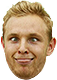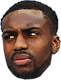Barça is bankrupt. 1.000M€ debt and January's wages can't be paid

Latest Spurs videos from Sky Sports

MemphisWill

The metric system is vastly superior, but that's not what I'm talking about here.
It's all mathematics, though, isn't it? My point being why would anyone listen to us on how to use mathematical punctuation when we still use a unit of measure ordained in the 12th century as 3 grains of barley taken from the middle of the ear.

When it comes to math, really, we Anglos should just always assume that its us who are the fools.

VirginiaSpur

Supporter
"Intelligent but stupid" - Ahab
It's all mathematics, though, isn't it? My point being why would anyone listen to us on how to use mathematical punctuation when we still use a unit of measure ordained in the 12th century as 3 grains of barley taken from the middle of the ear.

When it comes to math, really, we Anglos should just always assume that its us who are the fools.
In point of fact, systems of measurements are not math.

MemphisWill

In point of fact, systems of measurements are not math.
In point of fact, they are. Without systems of measurement, math is merely numbers. Math is a language, numbers are the alphabet of that language and systems of measurement are the root words that give meaning to arrangement of that alphabet.VirginiaSpur

Supporter
"Intelligent but stupid" - Ahab
In point of fact, they are. Without systems of measurement, math is merely numbers. Math is a language, numbers are the alphabet of that language and systems of measurement are the root words that give meaning to arrangement of that alphabet.
Systems of measurement are arbitrary. They are not math.

MemphisWill

Systems of measurement are arbitrary. They are not math.
Words are arbitrary, language is not. Without systems of measurement "math" is meaningless.

Supporter

VirginiaSpur

Supporter
"Intelligent but stupid" - Ahab
Words are arbitrary, language is not. Without systems of measurement "math" is meaningless.Who says that math has to be applied? Every single math teacher I ever had was dogshit at explaining why math was important to learn.

There are entire fields of mathematics devoted to pure math and number theory, exploring the limits of mathematics without applied constraints.

MemphisWillWho says that math has to be applied? Every single math teacher I ever had was dogshit at explaining why math was important to learn.

There are entire fields of mathematics devoted to pure math and number theory, exploring the limits of mathematics without applied constraints.
And people made up Klingon. Just because people do something, doesn't make it useful. Math without application is as useful as tits on a boar.

Further, numbers, themselves, are nothing more than units of measure. 1 is 10 0.1s. 5 is 5 1s. They're measuring abstract quantities, but they're still measuring that quantity.

Donki

The metric system is vastly superior, but that's not what I'm talking about here.
Could well be superior, but I cant see the shit kickers around my bit of gods green earth knowing how far a "country km" is.

VirginiaSpur

Supporter
"Intelligent but stupid" - Ahab
And people made up Klingon. Just because people do something, doesn't make it useful. Math without application is as useful as tits on a boar.
Further, numbers, themselves, are nothing more than units of measure. 1 is 10 0.1s. 5 is 5 1s. They're measuring abstract quantities, but they're still measuring that quantity.
This entire conversation is critically useful. I think everyone should be brought in to comment on this argument.

r-u-s-x

Colon as a delimiter vs semi colon is as frustrating as comma and fullstop.

Excel geeks in the house

Dr Rocktopus

🎃🦇🎃🦇🎃
Well if nothing else I've learnt that the application of mathematics was settled after some bloke met Rick. Whoever he is.

MemphisWill

This entire conversation is critically useful. I think everyone should be brought in to comment on this argument.VirginiaSpur

Supporter
"Intelligent but stupid" - Ahab
Excel geeks in the house
Here is a fun excel formula that I have developed which has contributed to my psychosis.

=IF(AND(H43<\$H\$37,I43<\$I\$37,J43<\$J\$37),\$G\$37,IF(AND(H43<\$H\$38,I43<\$I\$38,J43<\$J\$38),\$G\$38,IF(AND(H43<\$H\$39,I43<\$I\$39,J43<\$J\$39),\$G\$39,IF(AND(H43<\$H\$40,I43<\$I\$40,J43<\$J\$40),\$G\$40))))

That formula combines with other, similar formulas to feed variables into the formula below.

=IF(\$H\$32="Single",\$H\$44,K43&"T "&IF(\$H\$33="Clear",,IF(OR(\$H\$33="Gray",\$H\$33="Bronze"),\$H\$33,IF(\$H\$33="Clear",,"Q"&\$H\$33)))&" / "&L43&" FBD"&" / "&IF(\$H\$34="Clear",,IF(OR(\$H\$34="Gray",\$H\$34="Bronze"),\$H\$34,IF(\$H\$34="Clear",,"Q"&\$H\$34)))&" ")&K43&"T"

That formula generates a complete order string for an insulated glass lite which contains tempering information, Low-E choices, tint options, glass thicknesses, and spacer bar information to submit to a glass manufacturer.

Here is a fun excel formula that I have developed which has contributed to my psychosis.

=IF(AND(H43<\$H\$37,I43<\$I\$37,J43<\$J\$37),\$G\$37,IF(AND(H43<\$H\$38,I43<\$I\$38,J43<\$J\$38),\$G\$38,IF(AND(H43<\$H\$39,I43<\$I\$39,J43<\$J\$39),\$G\$39,IF(AND(H43<\$H\$40,I43<\$I\$40,J43<\$J\$40),\$G\$40))))

That formula combines with other, similar formulas to feed variables into the formula below.

=IF(\$H\$32="Single",\$H\$44,K43&"T "&IF(\$H\$33="Clear",,IF(OR(\$H\$33="Gray",\$H\$33="Bronze"),\$H\$33,IF(\$H\$33="Clear",,"Q"&\$H\$33)))&" / "&L43&" FBD"&" / "&IF(\$H\$34="Clear",,IF(OR(\$H\$34="Gray",\$H\$34="Bronze"),\$H\$34,IF(\$H\$34="Clear",,"Q"&\$H\$34)))&" ")&K43&"T"

That formula generates a complete order string for an insulated glass lite which contains tempering information, Low-E choices, tint options, glass thicknesses, and spacer bar information to submit to a glass manufacturer.Dr Rocktopus

🎃🦇🎃🦇🎃
Today I learned that mathematics ends in an 'S'.r-u-s-x

Here is a fun excel formula that I have developed which has contributed to my psychosis.

=IF(AND(H43<\$H\$37,I43<\$I\$37,J43<\$J\$37),\$G\$37,IF(AND(H43<\$H\$38,I43<\$I\$38,J43<\$J\$38),\$G\$38,IF(AND(H43<\$H\$39,I43<\$I\$39,J43<\$J\$39),\$G\$39,IF(AND(H43<\$H\$40,I43<\$I\$40,J43<\$J\$40),\$G\$40))))

That formula combines with other, similar formulas to feed variables into the formula below.

=IF(\$H\$32="Single",\$H\$44,K43&"T "&IF(\$H\$33="Clear",,IF(OR(\$H\$33="Gray",\$H\$33="Bronze"),\$H\$33,IF(\$H\$33="Clear",,"Q"&\$H\$33)))&" / "&L43&" FBD"&" / "&IF(\$H\$34="Clear",,IF(OR(\$H\$34="Gray",\$H\$34="Bronze"),\$H\$34,IF(\$H\$34="Clear",,"Q"&\$H\$34)))&" ")&K43&"T"

That formula generates a complete order string for an insulated glass lite which contains tempering information, Low-E choices, tint options, glass thicknesses, and spacer bar information to submit to a glass manufacturer.
Nice.

I work in finance, you would be surprised how many big money decisions are made based on spreadsheet formula similar to this. Often after the original author having left the company and no one really knowing what it does.

VirginiaSpur

Supporter
"Intelligent but stupid" - Ahab
Nice.

I work in finance, you would be surprised how many big money decisions are made based on spreadsheet formula similar to this. Often after the original author having left the company and no one really knowing what it does.
I understand that completely, because I've been trying to find someone willing to learn this crap and they all run screaming in horror from it.

And it just keeps getting more and more complex.

shempz

Supporter
Nice.

I work in finance, you would be surprised how many big money decisions are made based on spreadsheet formula similar to this. Often after the original author having left the company and no one really knowing what it does.

Sometimes it takes me ages to figure out exactly what I was trying to do on some of the formulae in my excel worksheets when I've not used them for a while.

r-u-s-x

I understand that completely, because I've been trying to find someone willing to learn this crap and they all run screaming in horror from it.

And it just keeps getting more and more complex.
I've started doing a lot more sql in recent years but in both instances (excel) I have been choosing to break things up into chunks and not monster formula so others can follow.

After trying to decipher people's formula and fixing things that are broken. I now have a "motto". It's not if we can do it but whether we should.